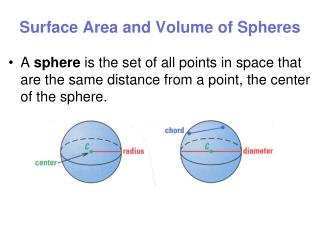DownloadDownload PresentationSurface Area and Volume of Spheres

# Surface Area and Volume of Spheres

Télécharger la présentation## Surface Area and Volume of Spheres

- - - - - - - - - - - - - - - - - - - - - - - - - - - E N D - - - - - - - - - - - - - - - - - - - - - - - - - - -
##### Presentation Transcript

1. Surface Area and Volume of Spheres • A sphere is the set of all points in space that are the same distance from a point, the center of the sphere.

2. Surface Area and Volume of Spheres • A radiusof a sphere is a segment from the center to a point on the sphere. • A chord of a sphere is a segment whose endpoints are on the sphere.

3. Surface Area and Volume of Spheres • A diameter is a chord that contains the center. As with all circles, the terms radius and diameter also represent distances, and the diameter is twice the radius.

4. More . . . • If a plane intersects a sphere, the intersection is either a single point or a circle. If the plane contains the center of the sphere, then the intersection is a great circleof the sphere. Every great circle of a sphere separates a sphere into two congruent halves called hemispheres.

5. Surface Area of a Sphere • Surface area = 4 π (radius)2 • S = 4 π r2 • Ex. of units: cm2, ft2, or m2 C Radius

6. Find the Surface Area of a Sphere • Find the surface area of the sphere. Round your answer to the nearest whole number. The radius is 8 in. 8 in.

7. Find the Surface Area of a Sphere • Find the surface area of the sphere. Round your answer to the nearest whole number. The radius is 5 cm. 10 cm.

8. Ex. 1: Comparing Surface Areas • Find the surface area. When the radius doubles, does the surface area double?

9. S = 4r2 = 4(2)2 = 16 in.2 S = 4r2 = 4(4)2 = 64 in.2 The surface area of the sphere in part (b) is four times greater than the surface area of the sphere in part (a) because 16 • 4 = 64 So, when the radius of a sphere doubles, the surface area DOES NOT double.

10. Ex. 2: Using a Great Circle • The circumference of a sphere (also referred to as the circumference of the great circle) is 13.8 feet. What is the surface area of the sphere?

11. Solution: Begin by finding the radius of the sphere. C = 2r 13.8 = 2r 13.8 = r (To solve for r, divide both sides by 2) 2 6.9 = r

12. Solution: Using a radius of 6.9 feet, the surface area is: S = 4r2 = 4(6.9)2 = 190.44 ft.2= 598.28 ft2 So, the surface area of the sphere is 190.44 feet squared.

13. Ex. 3: Finding the Surface Area of a Sphere

14. Volume of a Sphere radius C

15. Find the Volume of a Sphere • Find the volume of the sphere. Round your answer to the nearest whole number. a. 2 ft. Using your calculator, input: 4 Ab/c 3 2 ^ 3 =

16. Find the Volume of a Sphere a Find the volume of the hemisphere. Round your answer to the nearest whole number. Note: A hemisphere has HALF the volume of a sphere. • So simply use the volume formula, then ½ the answer. 5 in. Using your calculator, input: 4 Ab/c 3 5 ^ 3 = ≈523.95877 / 2 = ≈262

17. Find the Volume of a Sphere • Find the volume of the sphere. Write your answer in terms of . 6 in. To leave your answer in terms of pi… simply don’t input x 288 4 Ab/c 3 6 ^ 3 = Be sure to remember to include when you write your answer 

18. V = 4  r3 3 2 = 4  r3 3 6 = 4r3 1.5 = r3 1.14  r Formula for volume of a sphere. Substitute 2 for V. Multiply each side by 3. Divide each side by 4. Use a calculator to take the cube root. Last Example: Find r, if V = 2 3 2nd ^ 1 . 5 =